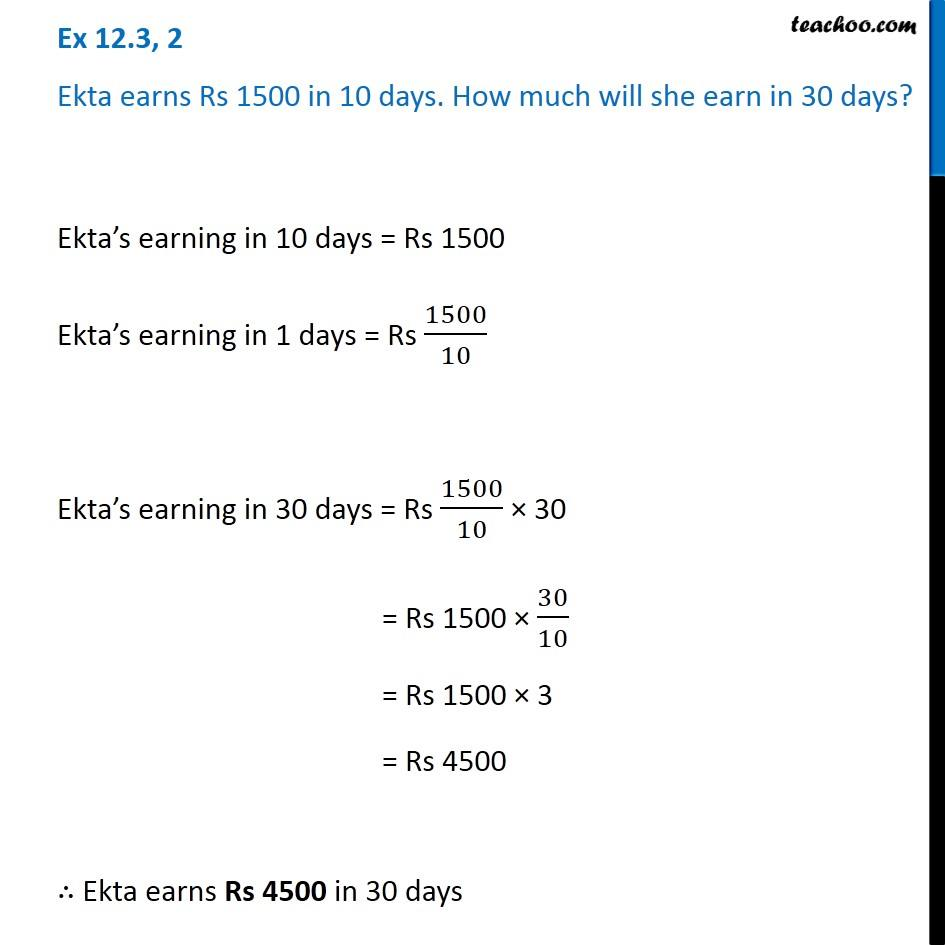1. Chapter 12 Class 6 Ratio And Proportion
2. Serial order wise
3. Ex 12.3

Transcript

Ex 12.3, 2 Ekta earns Rs 1500 in 10 days. How much will she earn in 30 days? Ekta’s earning in 10 days = Rs 1500 Ekta’s earning in 1 days = Rs 1500/10 Ekta’s earning in 30 days = Rs 1500/10 × 30 = Rs 1500 × 30/10 = Rs 1500 × 3 = Rs 4500 ∴ Ekta earns Rs 4500 in 30 days